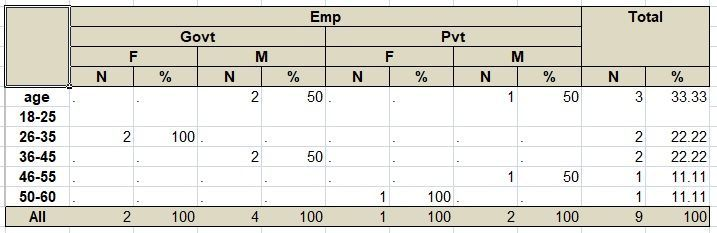## Percentage calculation in PROC TABULATE

Dear Experts,

I used Proc tabulate procedure to solve the tally information of the given (sample dataset) table.

But I required a tally of the Percentage columns as 100%. For more details kindly go through the below information.

``````data aaa;
input ID age\$ sex\$ Emp\$;
cards;
1 18-25 M Govt
2 50-60 F Pvt
6 26-35 F Govt
8 18-25 M Pvt
4 26-35 F Govt
5 36-45 M Govt
9 46-55 M Pvt
3 18-25 M Govt
0 36-45 M Govt
;
proc tabulate data = aaa;
class age sex Emp;
tables age all,Emp*(sex=' ' *(n*f=8. reppctn='%')) all='Total'*(n*f=8. reppctn='%')/rts=10;
run;``````

If you run the above code. It results N and % of 9 observations as Gender and Employment wise.

But I require a % column to calculate its value as 100% of its gender and employment like the Image given below.Kindly suggest the code which results in an image.

If you require any clarification. I will be glad to help.

1 ACCEPTED SOLUTION

Accepted Solutions

## Re: Percentage calculation in PROC TABULATE

You have used in your code REPPCT which is percentage per report.

You can use ROWPCTN to calculate row percentage or use PCTSUM to calculate column percentage.

2 REPLIES 2

## Re: Percentage calculation in PROC TABULATE

You have used in your code REPPCT which is percentage per report.

You can use ROWPCTN to calculate row percentage or use PCTSUM to calculate column percentage.

## Re: Percentage calculation in PROC TABULATE

Hi:
For purposes of completeness:
PCTN PCTSUM
ROWPCTN ROWPCTSUM
COLPCTN COLPCTSUM
REPPCTN REPPCTSUM
PAGEPCTN PAGEPCTSUM

are all the percent statistics. Using one of these depends on whether you want the percent of the count (N) or the percent of a numeric variable (PCTSUM). Look in the TABULATE documentation and note that TABULATE does an automatic multiply by 100, so you need to use a PICTURE format to get the % sign into the TABULATE output.

Cynthia
Discussion stats
• 2 replies
• 456 views
• 2 likes
• 3 in conversation